Latest Banking jobs   »   Quantitative Aptitude Quiz For IBPS RRB...

# Quantitative Aptitude Quiz For IBPS RRB PO, Clerk Prelims 2021- 27th July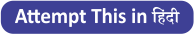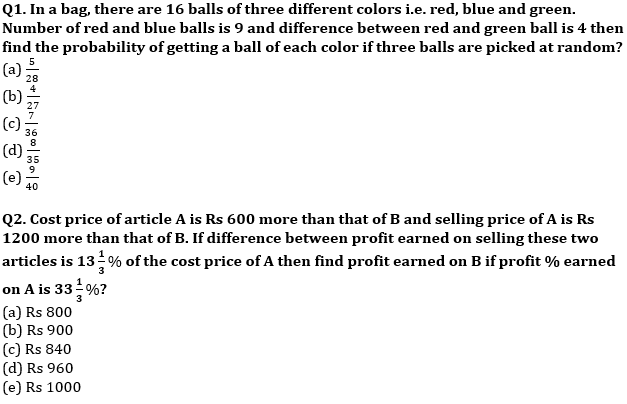Q3. In 100 lit mixture of milk and water, milk is 68 lit more than that of water. When ‘a’ lit of milk is taken out and (a+15) lit of water is added, milk becomes 50% more than that of water. Find value of ‘a’?
(a) 20
(b) 15
(c) 18
(d) 12
(e) 16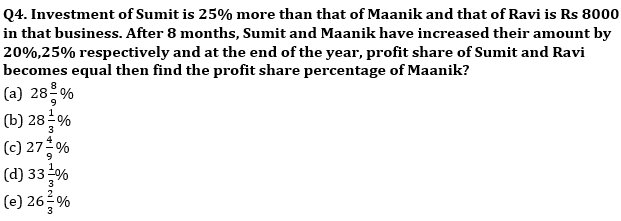Q5. Area of rectangle having length and breadth in the ratio of 4: 3 is 432 cm2. A square whose side is equal to diagonal of rectangle then find ratio of numerical value of perimeter to the area of square?
(a) 7:55
(b) 4:35
(c) None of these
(d) 1:8
(e) 2:15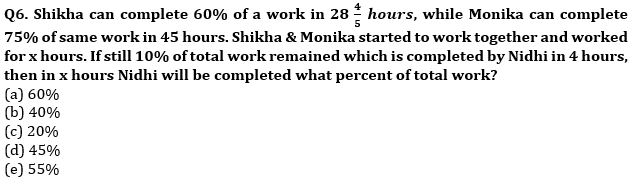Q7. A, B & C entered into a business by making investment in the ratio of 3 : 4 : 6 respectively. After one year A, B & C added Rs.100 , Rs.200 & Rs.300 respectively and after second year B & C withdrew Rs.100 & Rs.500 respectively. If at the end of three years B gets Rs.4050 as profit share out of total profit of Rs.12600, then find the investment done by C in second year?
(a) 1200 Rs.
(b) 1500 Rs.
(c) 1600 Rs.
(d)1800 Rs.
(e) 2100 Rs.

Q8. Ratio between length of two trains is 6 : 7 , while speeds of longer trains and smaller train are 72 km/hr & 108 km/hr respectively. If both trains crosses each other running is opposite direction in 10.4 sec, then find in what time longer train will be cross a platform whose length is 520 m ?
(a) 35sec
(b) 25 sec
(c) 45 sec
(d) 40 sec
(e) 5 5 sec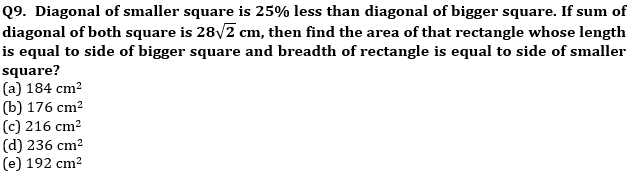Q10. Ratio between cost price and marked price of an article is 5 :7 and shopkeeper allows two successive discounts of 15% and 12% on marked price. If shopkeeper made a profit of Rs.354, then find at what price shopkeeper should sold the article to make a profit of 20%?
(a) 8400 Rs.
(b) 9600 Rs.
(c) 10200 Rs.
(d) 9000 Rs.
(e) 11600 Rs.

Q11. Veer lent Sameer Rs.24,000 on C.I. at the rate of 20% per annum and at the end of first year Sameer again borrowed Rs. X more from Veer on C.I. at the rate of 10% p.a.. If at the end of third year Sameer paid total amount of Rs. 55,992 to Veer, then find how much extra amount Sameer borrowed at the end of first year?
(a) 12000 Rs.
(b) 16000 Rs.
(c) 18000 Rs.
(d) 24000 Rs.
(e) 32000 Rs.

Q12. A shopkeeper mark up an A.C. 60% above its cost price and gives three successive discounts of 12½%, d% and 25%. If shopkeeper made a loss of 16% on AC then find the value of ‘d’?
(a) 25%
(b) 32%
(c) 24%
(d) 20%
(e) 15%

Q13. A 350 meters long train ‘A’ passed a pole in 17.5 sec and passed an another train ‘B’ running in the direction opposite to ‘A’ in 15.4 sec. If length of train ‘B’ is 420 meter, then in what time train ‘A’ will pass train ‘B’ when they both runs in same direction ?
(a) 72 sec
(b) 70 sec
(c) 77 sec
(d) 68 sec
(e) 65 sec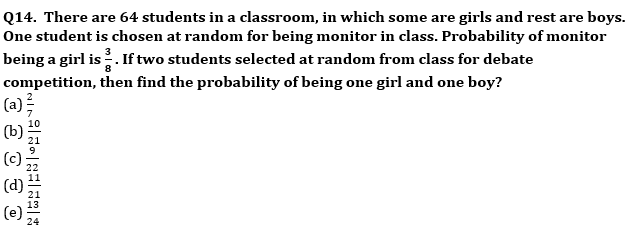Q15. In a River there are two boats P and Q, where boat P covers 120 km downstream and boat Q covers 120 km upstream. Boat Q takes equal time as boat P takes in covering the given distance. If sum of speed of boat P in still water and boat Q in still water is 64 km/hr and speed of water current is 4 km/hr then find the speed of boat Q in still water?
(a) 32 km/hr
(b) 36 km/hr
(c) 40 km/hr
(d) 42 km/hr
(e) 48 km/hr

Solutions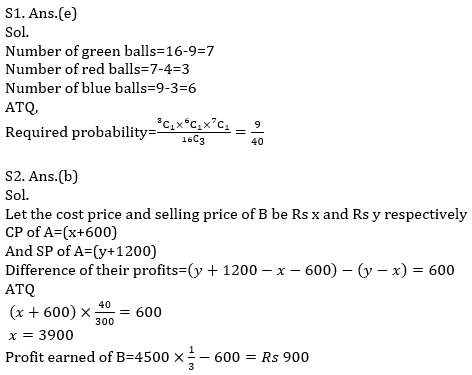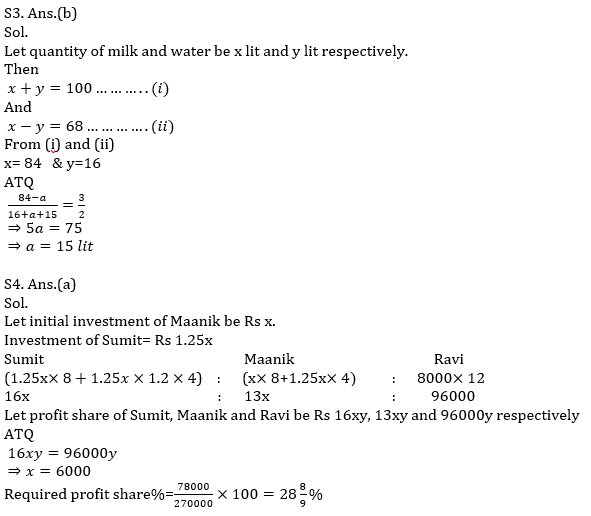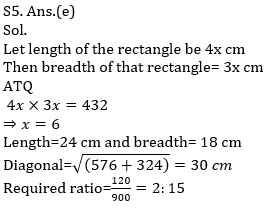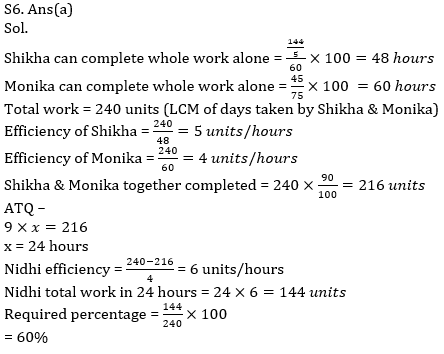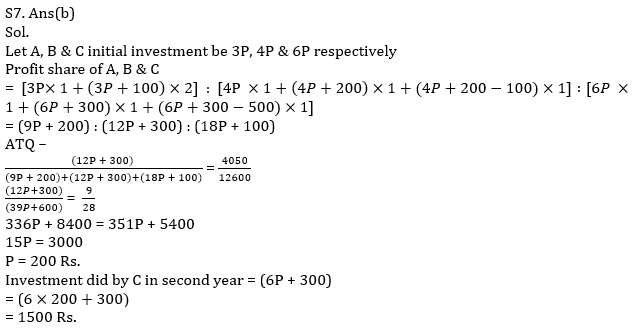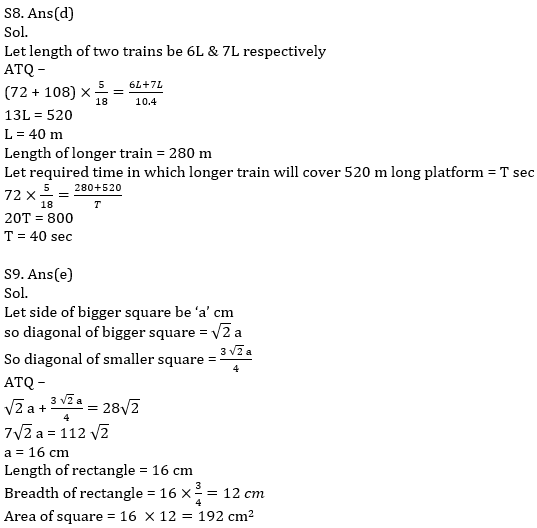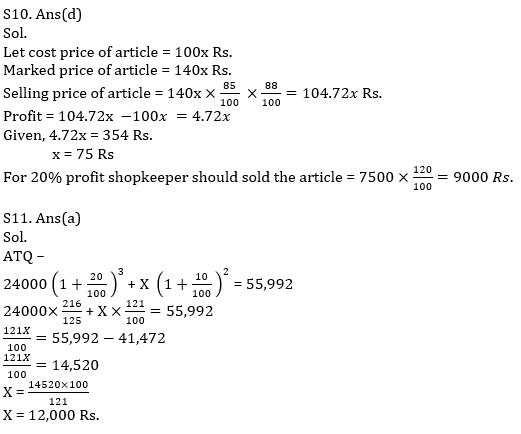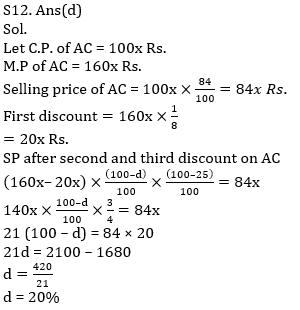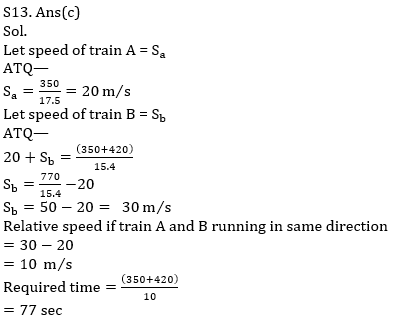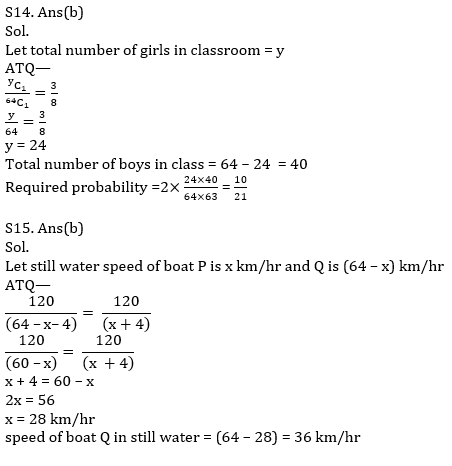#### Congratulations!